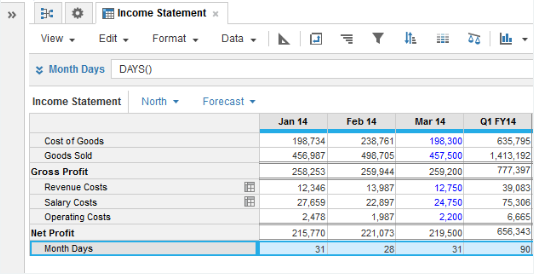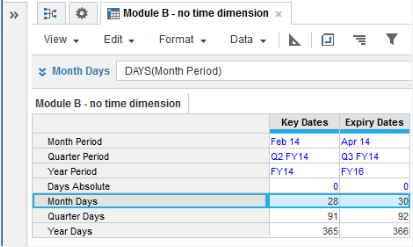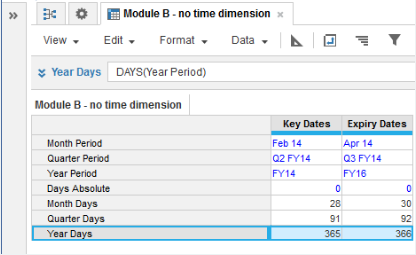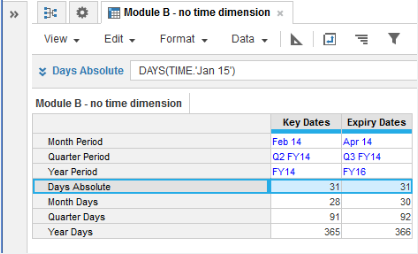1. Calculation functions
2. All Functions
3. Time and Date Functions
4. DAYSTakes no parameter or a single time period parameter. Returns the number of days in a time period:

• No parameter. Returns the number of days with reference to the source module time dimension.
• Time period parameter. Returns the number of days in the time period.
• Hard-coded. Returns the number of days with reference to the source timescale setting.

## Syntax

• `DAYS()`
• `DAYS(x)` where x is time period-formatted
• `DAYS(TIME.'Feb 15')` where a single hard-coded parameter makes reference to the timescale

## Format

Input Format Output Format

x: Time period

Number

## Arguments

The function uses the following arguments:

• x: (Optional) - Time period-formatted number, or a single hard-coded parameter that makes reference to the timescale

## Constraints

The function has the following constraints:

• If function takes a parameter, source must be time period-formatted.
• Result must be number-formatted.
• If time period is blank, result is zero.

## Example

• DAYS() where Month Days is numeric and references Jan 14 on the time dimension = 31:
•• DAYS(Month Period) where the result is numeric and Month Period source line item is time period-formatted for months and is Feb 14 = 28:
•• DAYS(Year Period) where the result is numeric and Year Period source line item is time period-formatted for years and is FY16= 366• DAYS(TIME.'Jan 15') where the result is numeric and the timescale includes Jan 15 = 31:
•